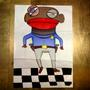1. Forum
2. >
3. Topic: French
4. >
5. "Quatre moins huit font ?"

# "Quatremoinshuitfont?"

## Translation:Four minus eight is?

January 2, 2013In English, we say "Four minus eight equals?" as opposed to "Four minus eight equal?," which is one of the alternatives offered as correct. We would include the "s" because we are not referring to the quantity of four equaling something, but rather to the equation.•570

It's a minor thing – but in English its usually stylistically frowned upon to use numerals and letters for number in the same sentence. You should write '4 minus 8' or 'four minus eight'They are not homophones. "mois" is pronounced [mwa] and "moins" [mwɛ̃], The last vowel in "mois" would be close to the one in "hat". The one in "moins" is a nasal, something you don't really have in English, but that might be close to the sound of "ain't" with a very strong southern US accent.•105

In many dialects of French, the pronunciation is much more distinct than Duolingo's.I said "Four minus eight makes what?" which would be a perfectly acceptable way of asking this question. But it wasn't accepted.four minus eight are? four or minus four is plural so 'are' not 'is.' Yes?In English you can say "What is four less THAN eight" which means "eight minus four". is there a French equivalent? "less than" doesn't mean the same as "minus"•54

why is it "makes" and not "make"?

The subject of the sentence is "Four minus eight".
This calculation makes.... This equation makes.....•1013

This makes no sense to me. Tricky way of teaching? ?•36

Four minus eight will be- means the same•105

But no English speaker would say it.

No, "will be" is future tense. This sentence is in present tense.Given that the topic is "time" and not a mathematical equation, the correct translation should be along the line of "Is it four to eight?" not four minus eight is? which if it were indeed an equation would be asked as, "What is four minus eight?"•676

Is it eight minutes to four? Is it 3:52? This is a question of what time it is, not an equation.If this is a mathematical question, it is a strange choice for people starting to learn French, as the answer would be "minus 4". What's next then? Algebra!